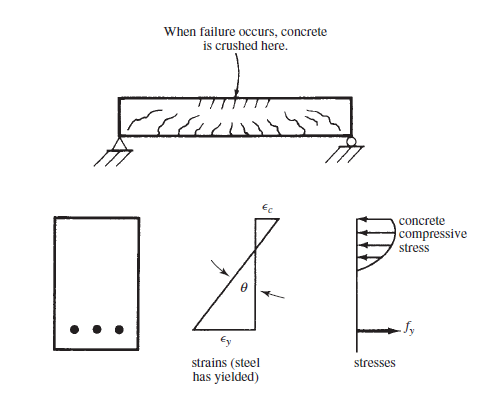### reinforced concrete beam behavior under load

if a reinforced concrete beam subjected to a small transverse load that increases gradually. under transverse load, the beam will go through three stages before the collapse. these stages are: uncracked concrete stage, concrete crack-elastic stresses stage and beam failure-ultimate stage.

• uncracked concrete stage: at the beginning of loading and when the load is less than the modulus of rupture(is the bending stress in which the beam start to crack).  in this stage, the beam will resist the compression stress and tensile stress with no cracks.  beam in figure 1 will be subjected to compression on top and tension in the bottom.
Figure 1

• concrete cracked elastic stress stage: once the load is more than the modulus of rapture. the beam will start to cracks in tension zone(bottom of the beam). as the load increase cracks will spread up near the neutral axis. and the neutral axis will begin to move up. concrete will crack in the location which the stress exceeds the modulus of rapture.or in other words where the moment exceeds the cracking moment( cracking moment is the moment at which the tensile stress is equal to the modulus of rupture). in this stage, concrete will not be able to resist tension anymore. reinforcing steel will resist tensile stress. this stage will continue as long the compressive stress in top of the beam is less than half of concrete compressive stress fc' and tensile stress in steel is less than yielding stress. in this stage, concrete stress will vary linearly as shown in figure 2. usually for a beam under service-load applied moment will exceed the cracking moment. the compressive stress will be less than 0.5fc'. therefore, concrete will cracks under service load and steel will resist tensile stress.

Figure 2

• Beam failure-ultimate strength stage: as the load increase and the compressive stress is more than 0.50*fc'. cracks will increase and move upward. similarly, the neutral axis will move upward. at this stage, steel will yield and the concrete compressive stress will change from a straight line to as shown in figure 3. diagram in figure 4 illustrating the relation between curvature θ and moment. where θ is the angle change of beam section over a certain length θ=ϵ/y. where ϵ is the strain of beam fiber at distance y. from the diagram, for the first stage and when the applied moment is less than Mcr. we can notice that strain is small and the curve is vertical. when the applied moment exceeds Mcr, the slope of curve decrease and the strain increased. in this stage, beam stiffness is less than the initial stage. the strain will increase sharply when the reinforcing steel yielded as shown in the diagram below. the beam will not fail suddenly when the steel yielded. the beam will be able to carry a small amount of moment before failure as shown in the diagram.Figure 3

Figure 4